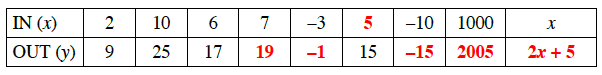### Home > CC3 > Chapter 4 > Lesson 4.1.2 > Problem4-19

4-19.

Copy and complete the table below. Homework Help ✎

 IN (x) 2 10 6 7 –3 –10 1000 x OUT (y) 9 25 17 15

Look for a pattern between the input numbers and output numbers.1. Explain in words what is done to the input value $(x)$ to produce the output value $(y)$.

Describe the pattern you found in the table in words.

2. Write the rule you described in part (a) with algebraic symbols.

Describe the pattern you found in the table in numbers.

What did you write in the OUT $(y)$ entry for the column in the IN $(x)$ row labeled $x$?